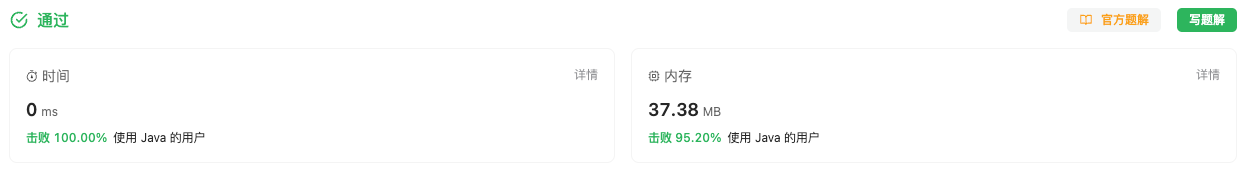#### 2525. 根据规则将箱子分类

###### 来源: 每日一题 2023.10.20

• 如果满足以下条件，那么箱子是 `"Bulky"` 的：

• 箱子 至少有一个 维度大于等于 `10^4`
• 或者箱子的 体积 大于等于 `10^9`
• 如果箱子的质量大于等于 `100` ，那么箱子是 `"Heavy"` 的。

• 如果箱子同时是 `"Bulky"``"Heavy"` ，那么返回类别为 `"Both"`

• 如果箱子既不是 `"Bulky"` ，也不是 `"Heavy"` ，那么返回类别为 `"Neither"`

• 如果箱子是 "Bulky" 但不是 "Heavy" ，那么返回类别为 `"Bulky"`

• 如果箱子是 `"Heavy`" 但不是 `"Bulky"` ，那么返回类别为 `"Heavy"`

``````输入：length = 1000, width = 35, height = 700, mass = 300

``````

``````输入：length = 200, width = 50, height = 800, mass = 50

``````

• `1 <= length, width, height <= 10^5`
• `1 <= mass <= 10^3`
``````class Solution {
public String categorizeBox(int length, int width, int height, int mass) {

}
}
``````

#### 分析与题解

• 花式整活→位运算

这个题目其实非常的简单, 根本不需要考虑, 按照题目的条件写逻辑分支语句即可. 但是我非要整活, 用`switch``状态机` 的思想, 位运算的 `按位与``按位或`, 来组合出当前盒子的状态.

这个原理可以参考 任意组合判断还在用Switch?位运算符了解一下~ 这篇博客.

我们发现盒子的任何状态都是由 `Bulky``Heavy` 来组合的. 那我们就先来确定这两个原子性的状态.

假设 `Bulky` 我们设定的值为 `1 << 1` , `Heavy` 我们设定的值为 `1 << 2`. 那么我们判断条件成立时, 通过 `按位或` 的形式添加到盒子状态 `boxState` 中即可.

``````// 首先组合两个基础状态
// 假设 "Bulky" == 1 << 1, "Heavy" == 1 << 2
// 现在有一个状态代表box的状态 boxState
int Bulky = 1 << 1;
int Heavy = 1 << 2;
int boxState = 0;
if(length >= 10000 || width >= 10000 || height >= 10000 || (long)length * width * height >= Math.pow(10, 9)) {
boxState |= Bulky;
}
if(mass >= 100) {
boxState |= Heavy;
}
``````

然后一个switch分支逻辑中, 按照不同的状态返回不同字符串即可.

``````switch(boxState) {
case 1<<1:
return "Bulky";
case 1<<2:
return "Heavy";
case (1<<1)|(1<<2):
return "Both";
default:
return "Neither";
}
``````

整体逻辑代码如下所示.

``````class Solution {
public String categorizeBox(int length, int width, int height, int mass) {
// 首先组合两个基础状态
// 假设 "Bulky" == 1 << 1, "Heavy" == 1 << 2
// 现在有一个状态代表box的状态 boxState
int Bulky = 1 << 1;
int Heavy = 1 << 2;
int boxState = 0;
if(length >= 10000 || width >= 10000 || height >= 10000 || (long)length * width * height >= Math.pow(10, 9)) {
boxState |= Bulky;
}
if(mass >= 100) {
boxState |= Heavy;
}
switch(boxState) {
case 1<<1:
return "Bulky";
case 1<<2:
return "Heavy";
case (1<<1)|(1<<2):
return "Both";
default:
return "Neither";
}

}
}
``````

复杂度分析:

• 时间复杂度: O(1), 常量级别的时间复杂度.
• 空间复杂度: O(1), 常量级别的空间复杂度.

结果如下所示.IT界无底坑洞栋主 欢迎加Q骚扰:676758285